Publicité

# Java Tutorial: Part 4 - Data and Calculations

16 Nov 2021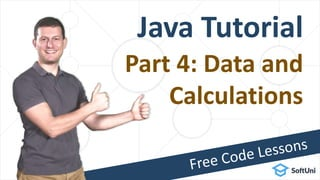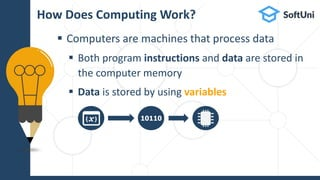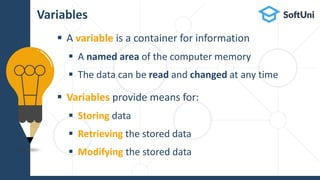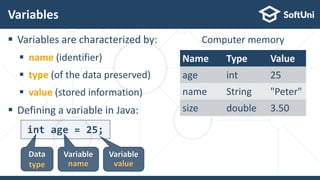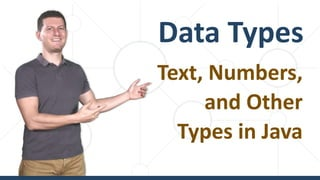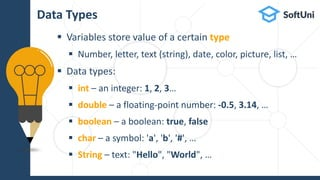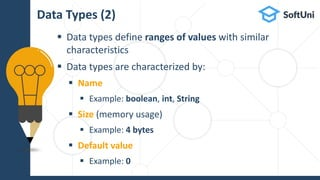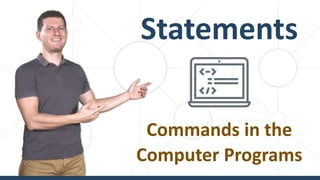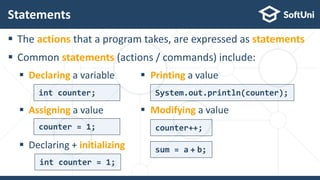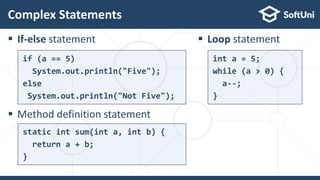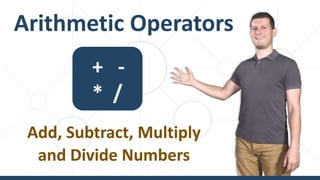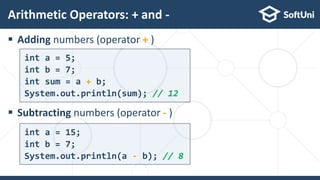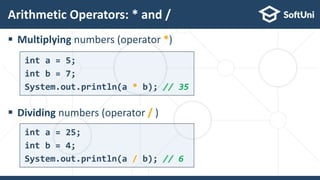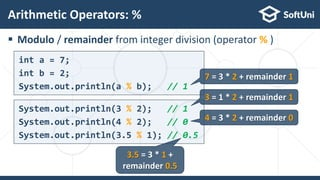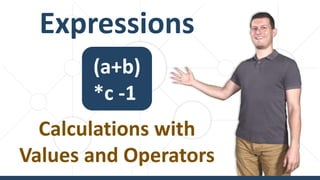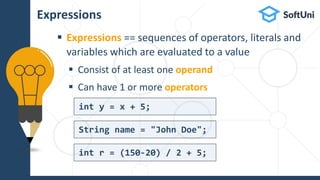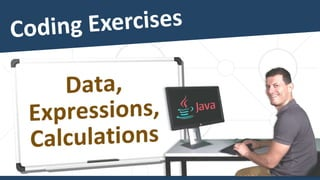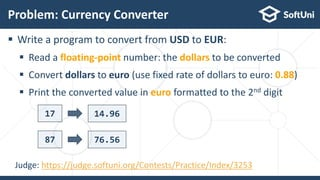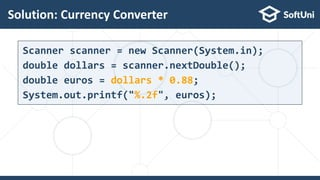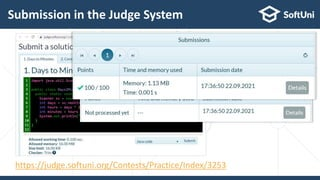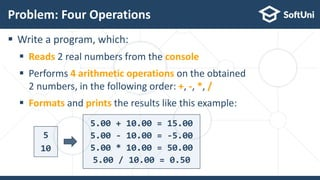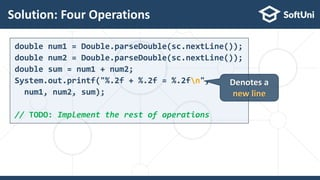1 sur 26
Publicité

### Java Tutorial: Part 4 - Data and Calculations

1. Java Tutorial Part 4: Data and Calculations
2. Variables Storing and Processing Data in the Memory
3.  Computers are machines that process data  Both program instructions and data are stored in the computer memory  Data is stored by using variables How Does Computing Work? 10110
4.  A variable is a container for information  A named area of the computer memory  The data can be read and changed at any time  Variables provide means for:  Storing data  Retrieving the stored data  Modifying the stored data Variables
5.  Variables are characterized by:  name (identifier)  type (of the data preserved)  value (stored information)  Defining a variable in Java: Variables int age = 25; Data type Variable name Variable value Name Type Value age int 25 name String "Peter" size double 3.50 Computer memory
6. Data Types Text, Numbers, and Other Types in Java
7.  Variables store value of a certain type  Number, letter, text (string), date, color, picture, list, …  Data types:  int – an integer: 1, 2, 3…  double – a floating-point number: -0.5, 3.14, …  boolean – a boolean: true, false  char – a symbol: 'a', 'b', '#', …  String – text: "Hello", "World", … Data Types
8.  Data types define ranges of values with similar characteristics  Data types are characterized by:  Name  Example: boolean, int, String  Size (memory usage)  Example: 4 bytes  Default value  Example: 0 Data Types (2)
9. Statements Commands in the Computer Programs
10.  The actions that a program takes, are expressed as statements  Common statements (actions / commands) include:  Declaring a variable  Assigning a value  Declaring + initializing Statements int counter; counter = 1; int counter = 1;  Printing a value System.out.println(counter);  Modifying a value counter++; sum = a + b;
11.  If-else statement  Method definition statement Complex Statements if (a == 5) System.out.println("Five"); else System.out.println("Not Five");  Loop statement int a = 5; while (a > 0) { a--; } static int sum(int a, int b) { return a + b; }
12. Arithmetic Operators Add, Subtract, Multiply and Divide Numbers + - * /
13.  Adding numbers (operator + )  Subtracting numbers (operator - ) Arithmetic Operators: + and - int a = 5; int b = 7; int sum = a + b; System.out.println(sum); // 12 int a = 15; int b = 7; System.out.println(a - b); // 8
14.  Multiplying numbers (operator *)  Dividing numbers (operator / ) Arithmetic Operators: * and / int a = 5; int b = 7; System.out.println(a * b); // 35 int a = 25; int b = 4; System.out.println(a / b); // 6
15.  When dividing integers, the result is also integer:  When dividing floating-points, the result is also floating-point: Division Behavior in Java int a = 25; System.out.println(a / 4); // Integer result: 6 System.out.println(a / 0); // Error: division by 0 double a = 15; System.out.println(a / 2.0); // 7.5 System.out.println(a / 0.0); // Infinity System.out.println(0 / 0.0); // NaN
16.  Modulo / remainder from integer division (operator % ) Arithmetic Operators: % int a = 7; int b = 2; System.out.println(a % b); // 1 System.out.println(3 % 2); // 1 System.out.println(4 % 2); // 0 System.out.println(3.5 % 1); // 0.5 7 = 3 * 2 + remainder 1 3 = 1 * 2 + remainder 1 4 = 3 * 2 + remainder 0 3.5 = 3 * 1 + remainder 0.5
17. Expressions Calculations with Values and Operators (a+b) *c -1
18.  Expressions == sequences of operators, literals and variables which are evaluated to a value  Consist of at least one operand  Can have 1 or more operators Expressions int r = (150-20) / 2 + 5; int y = x + 5; String name = "John Doe";
19.  Write a program to convert from USD to EUR:  Read a floating-point number: the dollars to be converted  Convert dollars to euro (use fixed rate of dollars to euro: 0.88)  Print the converted value in euro formatted to the 2nd digit Problem: Currency Converter 17 14.96 87 76.56 Judge: https://judge.softuni.org/Contests/Practice/Index/3253
20. Creating a New Project in IntelliJ IDEA
21. Solution: Currency Converter Scanner scanner = new Scanner(System.in); double dollars = scanner.nextDouble(); double euros = dollars * 0.88; System.out.printf("%.2f", euros);
22. Submission in the Judge System https://judge.softuni.org/Contests/Practice/Index/3253
23.  Write a program, which:  Reads 2 real numbers from the console  Performs 4 arithmetic operations on the obtained 2 numbers, in the following order: +, -, *, /  Formats and prints the results like this example: Problem: Four Operations 5 10 5.00 + 10.00 = 15.00 5.00 - 10.00 = -5.00 5.00 * 10.00 = 50.00 5.00 / 10.00 = 0.50
24. Solution: Four Operations double num1 = Double.parseDouble(sc.nextLine()); double num2 = Double.parseDouble(sc.nextLine()); double sum = num1 + num2; System.out.printf("%.2f + %.2f = %.2fn", num1, num2, sum); // TODO: Implement the rest of operations Denotes a new line
25.  …  …  … Next Steps  Join the SoftUni "Learn To Code" Community  Access the Free Coding Lessons  Get Help from the Mentors  Meet the Other Learners https://softuni.org

### Notes de l'éditeur

1. Hello, I am Svetlin Nakov from SoftUni (the Software University). I am here for the fourth part of my free Java coding tutorial – a series of video lessons with hands-on coding exercises. Today I continue with the fourth part of my free Java coding tutorial for absolute beginners. If you missed the previous parts, please review them first, to catch up. In this lesson, I will talk about variables and data types (such as string, integer number, floating-point number, boolean and others), statements (which define the commands in the programs), the most used arithmetic operators (plus, minus, multiply, divide and remainder) and the expressions in Java (or how to combine operators with values to implement a calculation). As usually, I will show you how to solve several coding problems and how to submit your solutions in the judge system for automated grading. Don't skip the coding exercises at the end of this lesson! They give you skills and coding experience. To learn coding, you should code! That's it! Let's start! Let's learn how to use data and calculations in Java.
2. Let's start with variables. In programming variables are used to store and process data in the in the computer memory. Variables are named memory areas, which hold data of certain type, like number of text. Let's learn more about them.
3. In the next section, I will give you a brief explanation of data types in programming and how they work in Java. I will mention the number types (int and double), the text type (String), the character type (char) and the Boolean type. And of course, I will demonstrate you these data types in a live coding demo.
4. In the next section I will explain the concept of "statements" in programming and the different types of statements.
5. Let's review the most important arithmetic operators, used to perform calculations with data in Java. I will explain the operators plus (for adding numbers), minus (for subtracting numbers), asterisk (for multiplying number), slash (for dividing numbers) and percent (for calculating the reminder of а division).
6. Now it's time for the hands-on exercises, because we want to learn skills, not just talk or watch video lessons. Follow the exercises: solve the practical problems and send your solutions to the judge system for grading. Learn by doing! Write code, run the code, test the code, make mistakes, fix them, run and test again, finally submit your code in the judge. This is how you learn coding: by practice. You will find the problem descriptions and the link to the judge system at softuni.org. Let's do the exercises. Let's code. Let's solve a few practical problems.
7. Did you like this code lesson? Do you want more? Subscribe to my YouTube channel to get more free video tutorials on computer programming and software engineering. Join the learners' community at softuni.org. Get free access to the practical exercises and the automated judge system for this code lesson. Get free help from mentors and meet other learners. Join now! SOFTUNI.ORG
Publicité